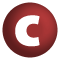# WithFilter

#### class WithFilter extends AnyRef

A class supporting filtered operations. Instances of this class are returned by method `withFilter`.

definition classes: TraversableLike

1. AnyRef
2. Any

### Value Members

1. #### def equals(arg0: Any): Boolean

This method is used to compare the receiver object (`this`) with the argument object (`arg0`) for equivalence

This method is used to compare the receiver object (`this`) with the argument object (`arg0`) for equivalence.

The default implementations of this method is an equivalence relation:

• It is reflexive: for any instance `x` of type `Any`, `x.equals(x)` should return `true`.
• It is symmetric: for any instances `x` and `y` of type `Any`, `x.equals(y)` should return `true` if and only if `y.equals(x)` returns `true`.
• It is transitive: for any instances `x`, `y`, and `z` of type `AnyRef` if `x.equals(y)` returns `true` and `y.equals(z)` returns `true`, then `x.equals(z)` should return `true`.

If you override this method, you should verify that your implementation remains an equivalence relation. Additionally, when overriding this method it is often necessary to override `hashCode` to ensure that objects that are "equal" (`o1.equals(o2)` returns `true`) hash to the same `Int` (`o1.hashCode.equals(o2.hashCode)`).

arg0

the object to compare against this object for equality.

returns

`true` if the receiver object is equivalent to the argument; `false` otherwise.

definition classes: AnyRef ⇐ Any
2. #### def flatMap[B, That](f: (A) ⇒ Traversable[B])(bf: CanBuildFrom[Repr, B, That]): That

Builds a new collection by applying a function to all elements of the outer traversable collection containing this `WithFilter` instance that satisfy predicate `p` and concatenating the results

Builds a new collection by applying a function to all elements of the outer traversable collection containing this `WithFilter` instance that satisfy predicate `p` and concatenating the results.

B

the element type of the returned collection.

That

the class of the returned collection. Where possible, `That` is the same class as the current collection class `Repr`, but this depends on the element type `B` being admissible for that class, which means that an implicit instance of type `CanBuildFrom[Repr, B, That]` is found.

f

the function to apply to each element.

bf

an implicit value of class `CanBuildFrom` which determines the result class `That` from the current representation type `Repr` and and the new element type `B`.

returns

a new collection of type `That` resulting from applying the given collection-valued function `f` to each element of the outer traversable collection that satisfies predicate `p` and concatenating the results.

3. #### def foreach[U](f: (A) ⇒ U): Unit

Applies a function `f` to all elements of the outer traversable collection containing this `WithFilter` instance that satisfy predicate `p`

Applies a function `f` to all elements of the outer traversable collection containing this `WithFilter` instance that satisfy predicate `p`.

U

the type parameter describing the result of function `f`. This result will always be ignored. Typically `U` is `Unit`, but this is not necessary.

f

the function that is applied for its side-effect to every element. The result of function `f` is discarded.

4. #### def hashCode(): Int

Returns a hash code value for the object

Returns a hash code value for the object.

The default hashing algorithm is platform dependent.

Note that it is allowed for two objects to have identical hash codes (`o1.hashCode.equals(o2.hashCode)`) yet not be equal (`o1.equals(o2)` returns `false`). A degenerate implementation could always return `0`. However, it is required that if two objects are equal (`o1.equals(o2)` returns `true`) that they have identical hash codes (`o1.hashCode.equals(o2.hashCode)`). Therefore, when overriding this method, be sure to verify that the behavior is consistent with the `equals` method.

definition classes: AnyRef ⇐ Any
5. #### def map[B, That](f: (A) ⇒ B)(bf: CanBuildFrom[Repr, B, That]): That

Builds a new collection by applying a function to all elements of the outer traversable collection containing this `WithFilter` instance that satisfy predicate `p`

Builds a new collection by applying a function to all elements of the outer traversable collection containing this `WithFilter` instance that satisfy predicate `p`.

B

the element type of the returned collection.

That

the class of the returned collection. Where possible, `That` is the same class as the current collection class `Repr`, but this depends on the element type `B` being admissible for that class, which means that an implicit instance of type `CanBuildFrom[Repr, B, That]` is found.

f

the function to apply to each element.

bf

an implicit value of class `CanBuildFrom` which determines the result class `That` from the current representation type `Repr` and and the new element type `B`.

returns

a new collection of type `That` resulting from applying the given function `f` to each element of the outer traversable collection that satisfies predicate `p` and collecting the results.

6. #### def toString(): String

Returns a string representation of the object

Returns a string representation of the object.

The default representation is platform dependent.

definition classes: AnyRef ⇐ Any
7. #### def withFilter(q: (A) ⇒ Boolean): WithFilter

Further refines the filter for this traversable collection

Further refines the filter for this traversable collection.

q

the predicate used to test elements.

returns

an object of class `WithFilter`, which supports `map`, `flatMap`, `foreach`, and `withFilter` operations. All these operations apply to those elements of this traversable collection which satify the predicate `q` in addition to the predicate `p`.